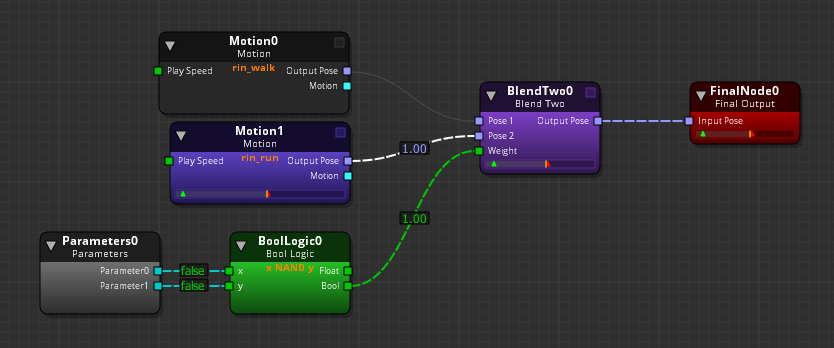Version:

# Using Math Nodes

O3DE’s Animation Editor features a set of math nodes that perform math operations on various types of input. The math nodes pass on the result(s) of the operation as output.

Topics

## Rotation Math 2 Node

With the Rotation Math 2 node, you can apply math operations to input rotations, which are represented by quaternions.

This node multiplies the input rotation and the specified Default Value to express an output rotation. The Default Value specifies the rotation of an unconnected input. This rotation value is expressed in Euler angle degrees (rotation about the X, Y, and Z axes).To use the Rotation Math 2 node

1. Connect the Output Rotation output rotation output(s) to the input(s) of the RotationMath2 node.

2. Select the RotationMath2 node.

3. In the right pane, on the Attributes tab,specify the Math Function. You can specify the following:. Rotate Multiplies two input quaternions or one input with the Default Value. Inverse rotate Multiplies the x input with the inverse of the y input. You can also use this to calculate the relative rotation of X with respect to Y.

4. If only one input rotation exists, specify the unconnected rotation values (X, Y, Z) for the Default Value.

## Rotation Limit Node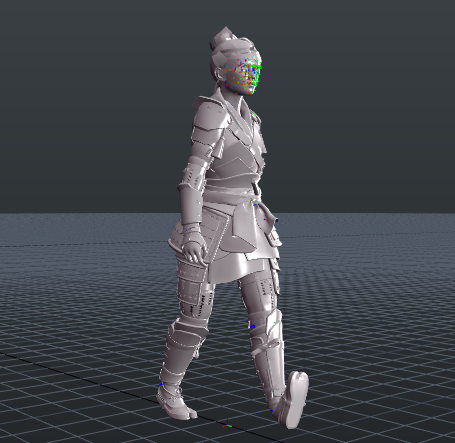With the Rotation Limit node, you can limit an input rotation. To do this, the node decomposes the rotations of the quaternion along relevant axes and limits their angles to a range. You define the minimum and the maximum possible angle values to remove ambiguity between the shortest or longest path angle defined by two values.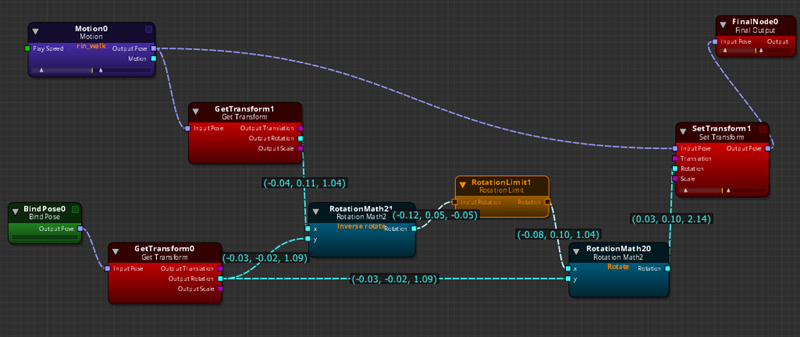To use the Rotation Limit node

1. Connect a rotation output to the Input Rotation of the RotationLimit node.

2. Select the RotationLimit node.

3. IIn the right pane, on the Attributes tab for Rotation limits, enter Min angle and Max angle values for X, Y, and Z.

Note:
The Min angle can’t exceed the Max angle. If it does, the error is displayed in red and the value doesn’t commit to the graph.
4. For Twist axis, select the X, Y, or Z Axis.

The Twist axis specifies which axis the Rotation Limit node is to decompose and apply edited constraints.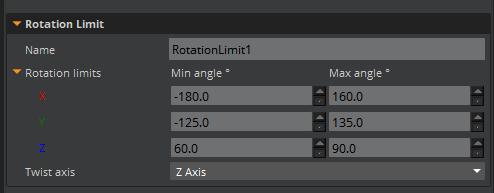## Vector Decompose Nodes

Using Vector Decompose nodes, you can output one or more specific values of a vector.

Example You have a 3D vector XYZ that indicates a position in a 3D world, but you only need its height (Z) for a computation. You would input your vector into a Vector3Decompose node and use only the Z output for your calculation.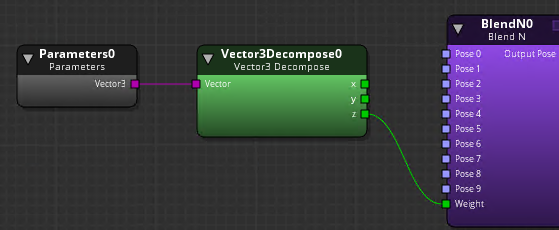You don’t need to use Vector Decompose nodes if you are simply adding or subtracting one of the X, Y, Z, or W positions. For typical Vector3 to Vector2 (and vice versa) conversion, or Vector3 to Vector4 (and vice versa) conversion, the Animation Editor automatically converts vectors in the following way:

• Vector2 to Vector3 - Adds the Z component set to 0.
• Vector3 to Vector2 - Ignores the Z component from Vector3.
• Vector3 to Vector4 - Adds the W component set to 0.
• Vector4 to Vector3 - Ignores the W component from Vector4.

## Boolean Logic Node

Using the Boolean Logic node, you can apply a function to two boolean inputs. Boolean values are always 1 or 0 (true or false), such as a check box item. The Boolean Logic node sees any non-zero value as true (1) and any zero value as false (0). For example, values 0.54, 10.43 or -2.25 are all true (1). Only 0.0 values are false (0).

When choosing an output type, you can output from the Float output or the Bool output. The Bool output passes on a 0 or 1. The Float output passes on a float value that you specify in the attributes.### Boolean Logic Node AttributesThe Boolean Logic node features a set of attributes that performs operations on boolean values.

AttributeDescription
NameName of the node.
Logic FunctionYou can set the following functions on boolean inputs:
Default ValueUsed as a second value when only one input value is specified.
Float Result When TrueSets a float value to output when the result of the boolean function is true (1). You must also use the Float output connector to output this value.
Float Result When FalseSets a float value to output when the result of the boolean function is false (0). You must also use the Float output connector to output this value.